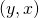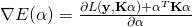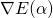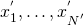# Non-Linear Classification Methods in Spark

In a previous post I covered how to apply classical linear estimators like support vector machines or logistic regression to a non-linear dataset using the kernel method. This article can be considered as a second part. While the previous article concerns about the theoretical foundation this article is about implementation issues and examples.

## 1. Model fitting

Again consider multivariate data given by a pairwhere the explanatory variableand the group coding. In the following we assume an iid. sample of sizegiven by. Starting where the previous post ends our aim is to minimize

(1)hereandis a certain penalty term.is aMatrix derived from a kernel function, a kernel can be understood as a function that measures a certain relation betweenand(e.g. spacial location). An important role is played by the choice of, it determines which method (eg. SVM, logit) is used. In general the problem lacks of an closed form solution, therefore we approximate the solution using the Newton-Raphson algorithm.

## 2. Newton-Raphson algorithm

For a given loss functionlet the gradient error function be given byand the Hessian. The Newton-Raphson algorithm is then to start with some initial estimateand updateuntil convergence.

### 2.1 Examples

#### 2.1.1 Ridge Regression

To construct a ridge regression usingwe have to choose the squared loss functionand thus

(2)using Newton-Raphson notice we getand. Starting with(3)Indeed, for the Ridge Regression case we can derive also an optimal solution analytically by simple taking the derivativesand equating them to zero which gives the same solution as the Newton-Raphson algorithm in one step. Fitted values are thus given by.

#### 2.1.2 Logistic Regression

To construct a logistic regressionhas to be chosen as. This lacks of an closed form solution, therefore we approximate the solution using the Newton-Raphson algorithm. Recall that we aim to minimizeThereforeand the Hessian is given by.

### 2.1 Computation

A computational problem using Newton-Raphson is to derive the Hessian sincecells has to be computed. A commonly used strategy is thus to approximate the Hessian for example using. Such Methods are called quasi-newton Methods. The Spark implementation to derive an support vector machine fitting for example make use of the L-BFGS method. Logit and Ridge Regression fitting rely on Stochastic gradient descent which does not use the Hessian at all and even approximates the gradient error function.

## 3. Prediction

Suppose a given fitted modelfitted using a training set of pairs. Consider another set of datawhich is often called test sample. In order to to reach a decision to which group these variables belong we use. This also gives a intuitive explanation how the prediction using the kernel method works. If observed test datais close, in the kernel sense, to the training datathan these two observations likely belong to the same group.

## 4. Implementation

The procedure is implemented in pySpark using the spark functions SVMWithSGD, LogisticRegressionWithLBFGS, LinearRegressionWithSGD. For curiosity also a Lasso implementation using LassoWithSGD was done, actually the model does not fit to the ones described above, because the error term looks different (L1 error norm). However using Lasso in the kernel context has an interesting effect, since lasso will not discard certain features but certain observations. To test the implementation train and test data is simulated using the following model:and with,where. The whole simulation is a bit long therefore it is available as a zeppelin notebook via github. For those who have no access to a zeppelin installation there exsits also a docker-compose setup including the notebook. As an example the code for the logit method is given above, for other linear methods the procedure is comparable.

import numpy as np
import matplotlib.pyplot as plt
import numpy as np
import matplotlib.pyplot as plt

return np.exp(-sum((x-y)**2)/(2*sigma**2))

def construct_K(Y,X,X_1,lamb):
sp_X=sc.parallelize(np.transpose(X)).zipWithIndex()
sp_X_1=sc.parallelize(np.transpose(X_1)).zipWithIndex()
sp_Y=sc.parallelize(Y).zipWithIndex().map(lambda(x,y) : (y,x) )
grid=sp_X.cartesian(sp_X_1)
return [sp_Y, K]

def construct_labeled(Y,K):
if type(acc) == list:
return (min(acc,x), acc + [x]  )
else:
return (min(acc,x), [acc] + [x]  )
jnd=Y.join(K).reduceByKey(lambda acc, x : add_element(acc,x) )
labeled=jnd.map(lambda(y,x) : LabeledPoint(x, x)  )
order=jnd.map(lambda (y,x): y)
return [labeled, order]

##Simualte the training sample
N=500
Y= np.random.randint(0,2,N)
degree=np.random.normal(0,1,N)*2*np.pi
X= [0+ (0.5 + Y*0.5)* np.cos(degree)+ np.random.normal(0,2,N)*0.05, 0 + (0.5 + Y*0.5)*np.sin(degree)+ np.random.normal(0,2,N)*0.05   ]

plt.scatter(X, X, c=Y)
plt.show()

#Example Logistic Regression

from pyspark.mllib.regression import LabeledPoint

Y_K=construct_K(Y,X,X,0.1)
l_train=construct_labeled(Y_K, Y_K)

# Evaluating the model on training data
from pyspark.mllib.classification import LogisticRegressionWithLBFGS, LogisticRegressionModel

LogitModel = LogisticRegressionWithLBFGS.train(l_train)
labelsAndPreds = l_train.map(lambda p: (p.label, LogitModel.predict(p.features)))
trainErr = labelsAndPreds.filter(lambda lp: lp != lp).count() / float(l_train.count())
print("Training Error = " + str(trainErr))

#Construct Test Sample and apply the model
##Generate data
#Simulation
N=200

Y_test=np.random.randint(0,2,N)
degree=np.random.normal(0,1,N)*2*np.pi
X_test=[0+ (0.5 + Y_test*0.5)* np.cos(degree)+ np.random.normal(0,2,N)*0.05, 0 + (0.5 + Y_test*0.5)*np.sin(degree)+ np.random.normal(0,2,N)*0.05]

#plot data
plt.scatter(X_test, X_test, c=Y_test)
plt.show()

Y_K_test=construct_K(Y_test,X_test,X,0.1)
l_test=construct_labeled(Y_K_test, Y_K_test)

##Logit
labelsAndPreds = l_test.map(lambda p: (p.label, LogitModel.predict(p.features)))
testErr = labelsAndPreds.filter(lambda lp: lp != lp).count() / float(l_train.count())
print("Prediction Error (Logit)= " + str(testErr))

#plot predictions
preds=labelsAndPreds.map(lambda lp: lp).collect()
sort_order=l_test.collect()
pred_sorted = [x for _,x in sorted(zip(sort_order,preds))]

plt.scatter(X_test, X_test, c=pred_sorted)
plt.show()


This site uses Akismet to reduce spam. Learn how your comment data is processed.